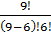### Sample Problem

Find the seventh term of (2a+b)9.

#### Solution

Recall that the (r+1)th term is nCrxn-ryr, so r is one less than the number of the term you are looking for. So, to find the 7th term, use r=6, n=9, x=2a, y=b:

9C6(2a)9-6b6=23a3b6=84(23)a3b6=672a3b6